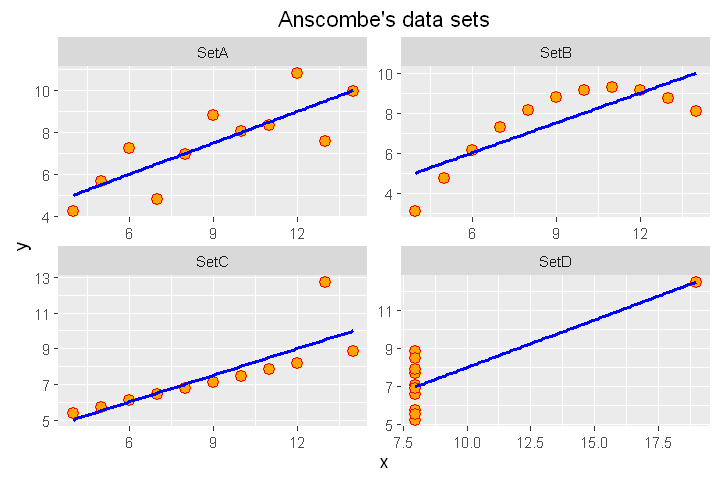# Fisseha Berhane, PhD

#### Data Scientist443-970-2353[email protected] CV Resume## The importance of Data Visualization¶

Before we perform any analysis and come up with any assumptions about the distributions of and relationships between variables in our datasets, it is always a good idea to visualize our data in order to understand their properties and identify appropriate analytics techniques. In this post, let's see the dramatic differences in conclutions that we can make based on (1) simple statistics only, and (2) data visualization.

The Anscombe dataset, which is found in the base R datasets packege, is handy for showing the importance of data visualization in data analysis. It consists of four datasets and each dataset consists of eleven (x,y) points.

#### The four datasets¶

In :
anscombe

x1x2x3x4y1y2y3y4
110.0010.0010.00 8.00 8.04 9.14 7.46 6.58
28.008.008.008.006.958.146.775.76
313.0013.0013.00 8.00 7.58 8.7412.74 7.71
49.009.009.008.008.818.777.118.84
511.0011.0011.00 8.00 8.33 9.26 7.81 8.47
614.0014.0014.00 8.00 9.96 8.10 8.84 7.04
76.006.006.008.007.246.136.085.25
8 4.00 4.00 4.0019.00 4.26 3.10 5.3912.50
912.0012.0012.00 8.0010.84 9.13 8.15 5.56
107.007.007.008.004.827.266.427.91
115.005.005.008.005.684.745.736.89

#### Let's make some massaging to make the data more convinient for analysis and plotting¶

Create four groups: setA, setB, setC and setD.

In :
library(ggplot2)
library(dplyr)
library(reshape2)

In :
setA=select(anscombe, x=x1,y=y1)
setB=select(anscombe, x=x2,y=y2)
setC=select(anscombe, x=x3,y=y3)
setD=select(anscombe, x=x4,y=y4)


Add a third column which can help us to identify the four groups.

In :
setA$group ='SetA' setB$group ='SetB'
setC$group ='SetC' setD$group ='SetD'

head(setA,4)  # showing sample data points from setA

xygroup
110 8.04SetA
28 6.95SetA
313 7.58SetA
49 8.81SetA

Now, let's merge the four datasets.

In :
all_data=rbind(setA,setB,setC,setD)  # merging all the four data sets
all_data[c(1,13,23,43),]  # showing sample

xygroup
110 8.04SetA
138 8.14SetB
2310 7.46SetC
438 7.91SetD

### Compare their summary statistics¶

In :
summary_stats =all_data%>%group_by(group)%>%summarize("mean x"=mean(x),
"Sample variance x"=var(x),
"mean y"=round(mean(y),2),
"Sample variance y"=round(var(y),1),
'Correlation between x and y '=round(cor(x,y),2)
)

models = all_data %>%
group_by(group) %>%
do(mod = lm(y ~ x, data = .)) %>%
do(data.frame(var = names(coef(.$mod)), coef = round(coef(.$mod),2),
group = .$group)) %>% dcast(., group~var, value.var = "coef") summary_stats_and_linear_fit = cbind(summary_stats, data_frame("Linear regression" = paste0("y = ",models$"(Intercept)"," + ",models\$x,"x")))

summary_stats_and_linear_fit

groupmean xSample variance xmean ySample variance yCorrelation between x and y Linear regression
1SetA 9 11 7.5 4.1 0.82 y = 3 + 0.5x
2SetB 9 11 7.5 4.1 0.82 y = 3 + 0.5x
3SetC 9 11 7.5 4.1 0.82 y = 3 + 0.5x
4SetD 9 11 7.5 4.1 0.82 y = 3 + 0.5x

If we look only at the simple summary statistics shown above, we would conclude that these four data sets are identical.

### What if we plot the four data sets?¶

In :
 ggplot(all_data, aes(x=x,y=y)) +geom_point(shape = 21, colour = "red", fill = "orange", size = 3)+
ggtitle("Anscombe's data sets")+geom_smooth(method = "lm",se = FALSE,color='blue') +
facet_wrap(~group, scales="free")As we can see from the figures above, the datasets are very different from each other. The Anscombe's quartet is a good example that shows that we have to visualize the relatonships, distributuions and outliers of our data and we shoul not rely only on simple statistics.

### Summary¶

We should look at the data graphically before we start analysis. Further, we should understand that basic statistics properties can often fail to capture real-world complexities (such as outliers, relationships and complex distributions) since summary statistics do not capture all of the complexities of the data.